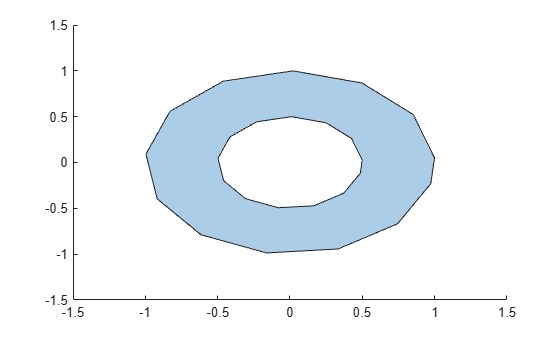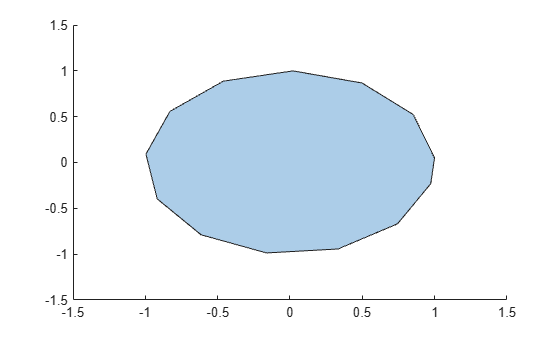# rmholes

Remove holes in `polyshape`

## Syntax

``polyout = rmholes(polyin)``

## Description

example

````polyout = rmholes(polyin)` returns a `polyshape` object made up of the solid boundaries of the polygon `polyin`, with all the hole boundaries removed.```

## Examples

collapse all

Create a polygon with a hole.

```t = 0.05:0.5:2*pi; x1 = cos(t); y1 = sin(t); x2 = 0.5*cos(t); y2 = 0.5*sin(t); polyin = polyshape({x1,x2},{y1,y2})```
```polyin = polyshape with properties: Vertices: [27x2 double] NumRegions: 1 NumHoles: 1 ```
`plot(polyin)`Create a new polygon with the hole removed.

`polyout = rmholes(polyin)`
```polyout = polyshape with properties: Vertices: [13x2 double] NumRegions: 1 NumHoles: 0 ```
`plot(polyout)`## Input Arguments

collapse all

Input `polyshape`, specified as a scalar, vector, matrix, or multidimensional array.

Data Types: `polyshape`

## Version History

Introduced in R2017b